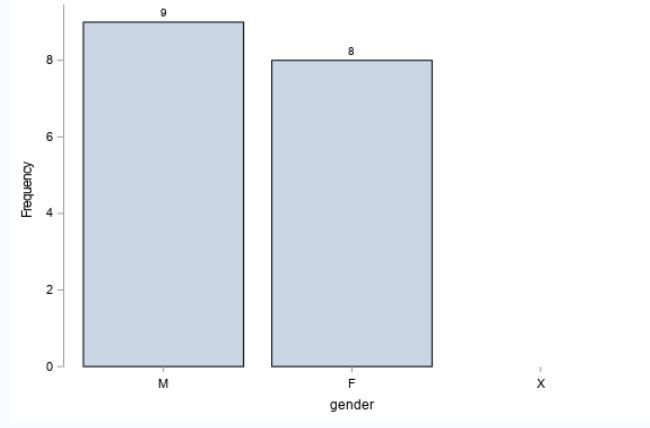## proc means - vbar - display freq=0

Hi,

As fas as I could read in the SAS online doc, it is currently not possible to display zero frequencies in a bar char.

I could manage to add it using the values options of xaxis statement but the value 0 is not displayed with datalabel and the bar at value zero is also not displayed.

Are you aware of any other solution I could consider (format?, other option? vbarbasic?, vbarparm?)

``````data cnt;
gender='M'; freq=9; output;
gender='F'; freq=8; output;
gender='X'; freq=0; output;
run;

proc print data=cnt;
run;

ods graphics / width=15cm height=10cm noborder;

proc sgplot data=cnt noborder;
vbar gender / freq=freq missing displaybaseline=off datalabel;
xaxis values=('M' 'F' 'X')
display = (noline);
run;

ods graphics off;``````1 ACCEPTED SOLUTION

Accepted Solutions

## Re: proc means - vbar - display freq=0

Here is how I would do it. Example using SASHELP.CLASS data set

``````proc format;
value \$ sexfmt "F"="Female"
"M"="Male"
"X"="X";
run;

proc summary data=sashelp.class nway completetypes;
class sex / preloadfmt order=formated missing;
format sex \$sexfmt.;
output out=counts;
run;

proc sgplot data=counts;
vbarparm category=sex response=_FREQ_ / nozerobars;
run;``````
3 REPLIES 3

## Re: proc means - vbar - display freq=0

Here is how I would do it. Example using SASHELP.CLASS data set

``````proc format;
value \$ sexfmt "F"="Female"
"M"="Male"
"X"="X";
run;

proc summary data=sashelp.class nway completetypes;
class sex / preloadfmt order=formated missing;
format sex \$sexfmt.;
output out=counts;
run;

proc sgplot data=counts;
vbarparm category=sex response=_FREQ_ / nozerobars;
run;``````

Thank you 🙂

## Re: proc means - vbar - display freq=0

Anytime 🙂

Discussion stats
• 3 replies
• 784 views
• 0 likes
• 2 in conversation